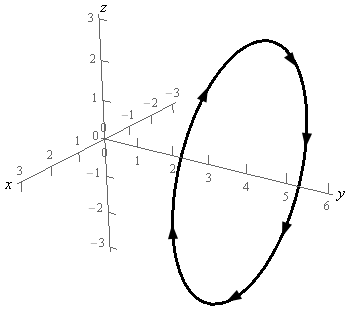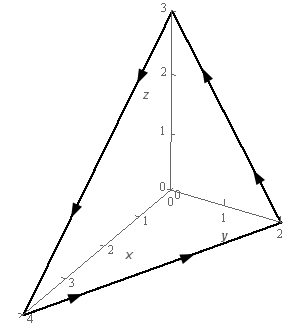Paul's Online Notes
Home / Calculus III / Surface Integrals / Stokes' Theorem
Show Mobile Notice Show All Notes Hide All Notes
Mobile Notice
You appear to be on a device with a "narrow" screen width (i.e. you are probably on a mobile phone). Due to the nature of the mathematics on this site it is best views in landscape mode. If your device is not in landscape mode many of the equations will run off the side of your device (should be able to scroll to see them) and some of the menu items will be cut off due to the narrow screen width.

### Section 17.5 : Stokes' Theorem

1. Use Stokes’ Theorem to evaluate $$\displaystyle \iint\limits_{S}{{{\mathop{\rm curl}\nolimits} \vec F\centerdot d\vec S}}$$ where $$\vec F = y\,\vec i - x\,\vec j + y{x^3}\,\vec k$$ and $$S$$ is the portion of the sphere of radius 4 with $$z \ge 0$$ and the upwards orientation. Solution
2. Use Stokes’ Theorem to evaluate $$\displaystyle \iint\limits_{S}{{{\mathop{\rm curl}\nolimits} \vec F\centerdot d\vec S}}$$ where $$\vec F = \left( {{z^2} - 1} \right)\,\vec i + \left( {z + x{y^3}} \right)\,\vec j + 6\,\vec k$$ and $$S$$ is the portion of $$x = 6 - 4{y^2} - 4{z^2}$$ in front of $$x = - 2$$ with orientation in the negative $$x$$-axis direction. Solution
3. Use Stokes’ Theorem to evaluate $$\displaystyle \int\limits_{C}{{\vec F\centerdot d\vec r}}$$ where $$\vec F = - yz\,\vec i + \left( {4y + 1} \right)\,\vec j + xy\,\vec k$$ and $$C$$ is is the circle of radius 3 at $$y = 4$$ and perpendicular to the $$y$$-axis. $$C$$ has a clockwise rotation if you are looking down the $$y$$-axis from the positive $$y$$-axis to the negative $$y$$-axis. See the figure below for a sketch of the curve.Solution
4. Use Stokes’ Theorem to evaluate $$\displaystyle \int\limits_{C}{{\vec F\centerdot d\vec r}}$$ where $$\vec F = \left( {3y{x^2} + {z^3}} \right)\,\vec i + {y^2}\,\vec j + 4y{x^2}\,\vec k$$ and $$C$$ is is triangle with vertices $$\left( {0,0,3} \right)$$, $$\left( {0,2,0} \right)$$ and $$\left( {4,0,0} \right)$$. $$C$$ has a counter clockwise rotation if you are above the triangle and looking down towards the $$xy$$-plane. See the figure below for a sketch of the curve.Solution